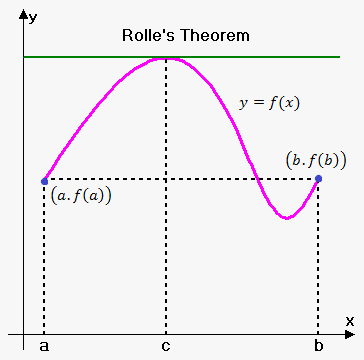# Rolle's Theorem

Rolle's Theorem. Suppose following three condition hold for function $y={f{{\left({x}\right)}}}$:

1. function is defined and continuous on closed interval ${\left[{a},{b}\right]}$;
2. exists finite derivative ${f{'}}{\left({x}\right)}$ on interval ${\left({a},{b}\right)}$;
3. ${f{{\left({a}\right)}}}={f{{\left({b}\right)}}}$.

then there exists point ${c}$ $\left({a}<{c}<{b}\right)$ such that ${f{'}}{\left({c}\right)}={0}$.Geometrically Rolle's theorem means the following: if ${f{{\left({a}\right)}}}={f{{\left({b}\right)}}}$ then there exists point ${c}$ at which tangent line is horizontal.

Note, that all three conditions are needed.

For function ${f{{\left({x}\right)}}}={x}-{\left[{x}\right]}$ on interval ${\left[{0},{1}\right]}$ first condition doesn't hold, because it is not continuous at ${x}={1}$. And ${f{'}}{\left({x}\right)}={1}$ on ${\left({0},{1}\right)}$, so there are no point ${c}$ from ${\left({0},{1}\right)}$ such that ${f{'}}{\left({c}\right)}={0}$.

For function ${f{{\left({x}\right)}}}={\left\{\begin{array}{c}{x}{\quad\text{if}\quad}{0}\le{x}\le\frac{{1}}{{2}}\\{1}-{x}{\quad\text{if}\quad}\frac{{1}}{{2}}\le{x}\le{1}\\ \end{array}\right.}$ second condition doesn't hold, because derivative doesn't exist at ${x}=\frac{{1}}{{2}}$. Aso ${f{'}}{\left({x}\right)}={1}$ on ${\left({0},\frac{{1}}{{2}}\right)}$ and ${f{'}}{\left({x}\right)}=-{1}$ on ${\left(\frac{{1}}{{2}},{1}\right)}$, so there are no point ${c}$ from ${\left({0},{1}\right)}$ such that ${f{'}}{\left({c}\right)}={0}$.

For function ${f{{\left({x}\right)}}}={x}$ on interval ${\left[{0},{1}\right]}$ third condition doesn't hold, ${f{{\left({0}\right)}}}\ne{f{{\left({1}\right)}}}$. And ${f{'}}{\left({x}\right)}={1}$ everywhere, so there are no point ${c}$ from ${\left({0},{1}\right)}$ such that ${f{'}}{\left({c}\right)}={0}$.